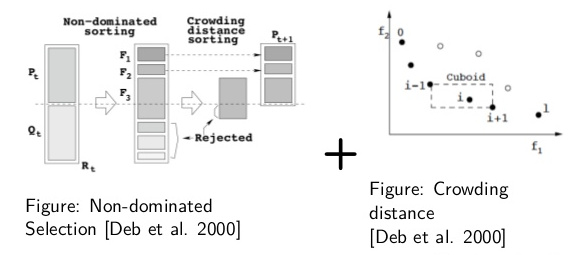# Non dominated sorting genetic algorithm (NSGA-II)

class pagmo::nsga2

Nondominated Sorting genetic algorithm II (NSGA-II)NSGA-II is a solid multi-objective algorithm, widely used in many real-world applications. While today it can be considered as an outdated approach, nsga2 has still a great value, if not as a solid benchmark to test against. NSGA-II genererates offsprings using a specific type of crossover and mutation and then selects the next generation according to nondominated-sorting and crowding distance comparison.

The version implemented in pagmo can be applied to box-bounded multiple-objective optimization. It also deals with integer chromosomes treating the last int_dim entries in the decision vector as integers.

See: Deb, K., Pratap, A., Agarwal, S., & Meyarivan, T. A. M. T. (2002). A fast and elitist multiobjective genetic algorithm: NSGA-II. IEEE transactions on evolutionary computation, 6(2), 182-197.

Public Types

typedef std::tuple<unsigned, unsigned long long, vector_double> log_line_type

Single entry of the log (gen, fevals, ideal_point)

typedef std::vector<log_line_type> log_type

The log.

Public Functions

nsga2(unsigned gen = 1u, double cr = 0.95, double eta_c = 10., double m = 0.01, double eta_m = 50., unsigned seed = pagmo::random_device::next())

Constructor.

Constructs the NSGA II user defined algorithm.

Parameters
• gen[in] Number of generations to evolve.

• cr[in] Crossover probability.

• eta_c[in] Distribution index for crossover.

• m[in] Mutation probability.

• eta_m[in] Distribution index for mutation.

• seed – seed used by the internal random number generator (default is random)

Throws

std::invalid_argument – if cr is not $$\in [0,1[$$, m is not $$\in [0,1]$$, eta_c is not in [1,100[ or eta_m is not in [1,100[.

population evolve(population) const

Algorithm evolve method.

Evolves the population for the requested number of generations.

Parameters

pop – population to be evolved

Throws

std::invalid_argument – if pop.get_problem() is stochastic, single objective or has non linear constraints. If int_dim is larger than the problem dimension. If the population size is smaller than 5 or not a multiple of 4.

Returns

evolved population

void set_seed(unsigned)

Sets the seed.

Parameters

seed – the seed controlling the algorithm stochastic behaviour

inline unsigned get_seed() const

Gets the seed.

Returns

the seed controlling the algorithm stochastic behaviour

inline void set_verbosity(unsigned level)

Sets the algorithm verbosity.

Sets the verbosity level of the screen output and of the log returned by get_log(). level can be:

• 0: no verbosity

• >0: will print and log one line each level generations.

Example (verbosity 1):

Gen:        Fevals:        ideal1:        ideal2:        ideal3:
1              0      0.0257554       0.267768       0.974592
2             52      0.0257554       0.267768       0.908174
3            104      0.0257554       0.124483       0.822804
4            156      0.0130094       0.121889       0.650099
5            208     0.00182705      0.0987425       0.650099
6            260      0.0018169      0.0873995       0.509662
7            312     0.00154273      0.0873995       0.492973
8            364     0.00154273      0.0873995       0.471251
9            416    0.000379582      0.0873995       0.471251
10            468    0.000336743      0.0855247       0.432144

Gen, is the generation number, Fevals the number of function evaluation used. The ideal point of the current population follows cropped to its 5th component.

Parameters

level – verbosity level

inline unsigned get_verbosity() const

Gets the verbosity level.

Returns

the verbosity level

void set_bfe(const bfe &b)

Sets the batch function evaluation scheme.

Parameters

b – batch function evaluation object

inline std::string get_name() const

Algorithm name.

Returns the name of the algorithm.

Returns

 std::string  containing the algorithm name

std::string get_extra_info() const

Extra info.

Returns extra information on the algorithm.

Returns

an  std::string  containing extra info on the algorithm

inline const log_type &get_log() const

Get log.

A log containing relevant quantities monitoring the last call to evolve. Each element of the returned std::vector is a nsga2::log_line_type containing: Gen, Fevals, ideal_point as described in nsga2::set_verbosity

Returns

an std::vector of nsga2::log_line_type containing the logged values Gen, Fevals, ideal_point